# Some topological and geometrical properties of new Banach sequence spaces

## Abstract

In the present paper, we introduce a new band matrix $\stackrel{ˆ}{F}$ and define the sequence space

${\ell }_{p}\left(\stackrel{ˆ}{F}\right)=\left\{x=\left({x}_{k}\right)\in \omega :\sum _{k}|\frac{{f}_{k}}{{f}_{k+1}}{x}_{k}-\frac{{f}_{k+1}}{{f}_{k}}{x}_{k-1}{|}^{p}<\mathrm{\infty };1\le p\le \mathrm{\infty }\right\},$

where ${f}_{k}$ is the k th Fibonacci number for every $k\in \mathbb{N}$. We also establish some inclusion relations concerning this space and determine its α-, β-, γ-duals. Further, we characterize some matrix classes on the space ${\ell }_{p}\left(\stackrel{ˆ}{F}\right)$ and examine some geometric properties of this space.

MSC:11B39, 46A45, 46B45, 46B20.

## 1 Introduction

Let ω be the space of all real-valued sequences. Any vector subspace of ω is called a sequence space. By ${\ell }_{\mathrm{\infty }}$, c, ${c}_{0}$ and ${\ell }_{p}$ ($1\le p<\mathrm{\infty }$), we denote the sets of all bounded, convergent, null sequences and p-absolutely convergent series, respectively. Also, we use the conventions that $e=\left(1,1,\dots \right)$ and ${e}^{\left(n\right)}$ is the sequence whose only non-zero term is 1 in the n th place for each $n\in \mathbb{N}$, where $\mathbb{N}=\left\{0,1,2,\dots \right\}$.

Let X and Y be two sequence spaces and $A=\left({a}_{nk}\right)$ be an infinite matrix of real numbers ${a}_{nk}$, where $n,k\in \mathbb{N}$. We write $A=\left({a}_{nk}\right)$ instead of $A={\left({a}_{nk}\right)}_{n,k=0}^{\mathrm{\infty }}$. Then we say that A defines a matrix mapping from X into Y and we denote it by writing $A:X\to Y$ if for every sequence $x={\left({x}_{k}\right)}_{k=0}^{\mathrm{\infty }}\in X$, the sequence $Ax={\left\{{A}_{n}\left(x\right)\right\}}_{n=0}^{\mathrm{\infty }}$, the A-transform of x, is in Y, where

${A}_{n}\left(x\right)=\sum _{k=0}^{\mathrm{\infty }}{a}_{nk}{x}_{k}\phantom{\rule{1em}{0ex}}\left(n\in \mathbb{N}\right).$
(1.1)

For simplicity in notation, here and in what follows, the summation without limits runs from 0 to ∞. Also, if $x\in \omega$, then we write $x=\left({x}_{k}\right)$ instead of $x={\left({x}_{k}\right)}_{k=0}^{\mathrm{\infty }}$.

By $\left(X,Y\right)$, we denote the class of all matrices A such that $A:X\to Y$. Thus, $A\in \left(X,Y\right)$ if and only if the series on the right-hand side of (1.1) converges for each $n\in \mathbb{N}$ and every $x\in X$ and we have $Ax\in Y$ for all $x\in X$.

The matrix domain ${X}_{A}$ of an infinite matrix A in a sequence space X is defined by

${X}_{A}=\left\{x=\left({x}_{k}\right)\in \omega :Ax\in X\right\}$
(1.2)

which is a sequence space.

The approach constructing a new sequence space by means of the matrix domain of a particular limitation method has recently been employed by several authors; see, for instance, .

Let Δ denote the matrix $\mathrm{\Delta }=\left({\mathrm{\Delta }}_{nk}\right)$ defined by

or

In the literature, the matrix domain ${\lambda }_{\mathrm{\Delta }}$ is called the difference sequence space whenever λ is a normed or paranormed sequence space. The idea of difference sequence spaces was introduced by Kızmaz . In 1981, Kızmaz  defined the sequence spaces

$X\left(\mathrm{\Delta }\right)=\left\{x=\left({x}_{k}\right)\in \omega :\left({x}_{k}-{x}_{k+1}\right)\in X\right\}$

for $X={\ell }_{\mathrm{\infty }}$, c and ${c}_{0}$. The difference space $b{v}_{p}$, consisting of all sequences $\left({x}_{k}\right)$ such that $\left({x}_{k}-{x}_{k-1}\right)$ is in the sequence space ${\ell }_{p}$, was studied in the case $0 by Altay and Başar  and in the case $1\le p\le \mathrm{\infty }$ by Başar and Altay  and Çolak et al. . The paranormed difference sequence space

$\mathrm{\Delta }\lambda \left(p\right)=\left\{x=\left({x}_{k}\right)\in \omega :\left({x}_{k}-{x}_{k+1}\right)\in \lambda \left(p\right)\right\}$

was examined by Ahmad and Mursaleen  and Malkowsky , where $\lambda \left(p\right)$ is any of the paranormed spaces ${\ell }_{\mathrm{\infty }}\left(p\right)$, $c\left(p\right)$ and ${c}_{0}\left(p\right)$ defined by Simons  and Maddox .

Recently, Başar et al.  have defined the sequence spaces $bv\left(u,p\right)$ and $b{v}_{\mathrm{\infty }}\left(u,p\right)$ by

$bv\left(u,p\right)=\left\{x=\left({x}_{k}\right)\in \omega :\sum _{k}|{u}_{k}\left({x}_{k}-{x}_{k-1}\right){|}^{{p}_{k}}<\mathrm{\infty }\right\}$

and

$b{v}_{\mathrm{\infty }}\left(u,p\right)=\left\{x=\left({x}_{k}\right)\in \omega :\underset{k\in \mathbb{N}}{sup}|{u}_{k}\left({x}_{k}-{x}_{k-1}\right){|}^{{p}_{k}}<\mathrm{\infty }\right\},$

where $u=\left({u}_{k}\right)$ is an arbitrary fixed sequence and $0<{p}_{k}\le H<\mathrm{\infty }$ for all $k\in \mathbb{N}$. These spaces are generalization of the space $b{v}_{p}$ for $1\le p\le \mathrm{\infty }$. Quite recently, Kirişçi and Başar  have introduced and studied the generalized difference sequence spaces

$\stackrel{ˆ}{X}=\left\{x=\left({x}_{k}\right)\in \omega :B\left(r,s\right)x\in X\right\}$

for $X={\ell }_{\mathrm{\infty }}$, ${\ell }_{p}$, c and ${c}_{0}$, where $1\le p<\mathrm{\infty }$ and $B\left(r,s\right)x=\left(s{x}_{k-1}+r{x}_{k}\right)$ ($r,s\ne 0$). Following Kirişçi and Başar , Sönmez  has examined the sequence space $X\left(B\right)$ as the set of all sequences whose $B\left(r,s,t\right)$-transforms are in the space $X\in \left\{{\ell }_{\mathrm{\infty }},{\ell }_{p},c,{c}_{0}\right\}$, where $B\left(r,s,t\right)$ denotes the triple band matrix $B\left(r,s,t\right)=\left\{{b}_{nk}\left(r,s,t\right)\right\}$ defined by

${b}_{nk}\left(r,s,t\right)=\left\{\begin{array}{cc}r\hfill & \left(n=k\right),\hfill \\ s\hfill & \left(n=k+1\right),\hfill \\ t\hfill & \left(n=k+2\right),\hfill \\ 0\hfill & \text{otherwise}\hfill \end{array}$

for all $n,k\in \mathbb{N}$ and $r,s,t\in \mathbb{R}-\left\{0\right\}$. Also in , the authors studied some difference sequence spaces.

In this paper, we define the Fibonacci difference matrix $\stackrel{ˆ}{F}$ by using the Fibonacci sequence ${\left\{{f}_{n}\right\}}_{n=0}^{\mathrm{\infty }}$ and introduce new sequence spaces ${\ell }_{p}\left(\stackrel{ˆ}{F}\right)$ and ${\ell }_{\mathrm{\infty }}\left(\stackrel{ˆ}{F}\right)$ related to the matrix domain of $\stackrel{ˆ}{F}$ in the sequence spaces ${\ell }_{p}$ and ${\ell }_{\mathrm{\infty }}$, respectively, where $1\le p<\mathrm{\infty }$. This study is organized as follows.

In Section 2, we give some notations and basic concepts including the Fibonacci sequence and a BK-space. In Section 3, we define a new band matrix with Fibonacci numbers and introduce the sequence spaces ${\ell }_{p}\left(\stackrel{ˆ}{F}\right)$ and ${\ell }_{\mathrm{\infty }}\left(\stackrel{ˆ}{F}\right)$. Also, we establish some inclusion relations concerning these spaces and construct the basis of the space ${\ell }_{p}\left(\stackrel{ˆ}{F}\right)$ for $1\le p<\mathrm{\infty }$. In Section 4, we determine the α-, β-, γ-duals of the spaces ${\ell }_{p}\left(\stackrel{ˆ}{F}\right)$ and ${\ell }_{\mathrm{\infty }}\left(\stackrel{ˆ}{F}\right)$. In Section 5, we characterize the classes $\left({\ell }_{p}\left(\stackrel{ˆ}{F}\right),X\right)$ and $\left({\ell }_{\mathrm{\infty }}\left(\stackrel{ˆ}{F}\right),X\right)$, where $1\le p<\mathrm{\infty }$ and X is any of the spaces ${\ell }_{\mathrm{\infty }}$, ${\ell }_{1}$, c and ${c}_{0}$. In the final section of the paper, we investigate some geometric properties of the space ${\ell }_{p}\left(\stackrel{ˆ}{F}\right)$ for $1.

## 2 The Fibonacci difference sequence space ${\ell }_{p}\left(\stackrel{ˆ}{F}\right)$

Define the sequence ${\left\{{f}_{n}\right\}}_{n=0}^{\mathrm{\infty }}$ of Fibonacci numbers given by the linear recurrence relations

${f}_{0}={f}_{1}=1\phantom{\rule{1em}{0ex}}\text{and}\phantom{\rule{1em}{0ex}}{f}_{n}={f}_{n-1}+{f}_{n-2},\phantom{\rule{1em}{0ex}}n\ge 2.$

Fibonacci numbers have many interesting properties and applications in arts, sciences and architecture. For example, the ratio sequences of Fibonacci numbers converges to the golden ratio which is important in sciences and arts. Also, some basic properties of Fibonacci numbers  are given as follows: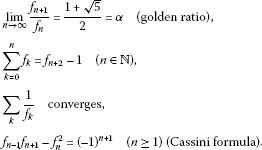Substituting for ${f}_{n+1}$ in Cassini’s formula yields ${f}_{n-1}^{2}+{f}_{n}{f}_{n-1}-{f}_{n}^{2}={\left(-1\right)}^{n+1}$.

A sequence space X is called a FK-space if it is a complete linear metric space with continuous coordinates ${p}_{n}:X\to \mathbb{R}$ ($n\in \mathbb{N}$), where denotes the real field and ${p}_{n}\left(x\right)={x}_{n}$ for all $x=\left({x}_{k}\right)\in X$ and every $n\in \mathbb{N}$. A BK space is a normed FK space, that is, a BK-space is a Banach space with continuous coordinates. The space ${\ell }_{p}$ ($1\le p<\mathrm{\infty }$) is a BK-space with ${\parallel x\parallel }_{p}={\left({\sum }_{k=0}^{\mathrm{\infty }}|{x}_{k}{|}^{p}\right)}^{1/p}$ and ${c}_{0}$, c and ${\ell }_{\mathrm{\infty }}$ are BK-spaces with ${\parallel x\parallel }_{\mathrm{\infty }}={sup}_{k}|{x}_{k}|$.

A sequence $\left({b}_{n}\right)$ in a normed space X is called a Schauder basis for X if for every $x\in X$, there is a unique sequence $\left({\alpha }_{n}\right)$ of scalars such that $x={\sum }_{n}{\alpha }_{n}{b}_{n}$, i.e., ${lim}_{m\to \mathrm{\infty }}\parallel x-{\sum }_{n=0}^{m}{\alpha }_{n}{b}_{n}\parallel =0$.

The α-, β- and γ-duals of the sequence space X are respectively defined by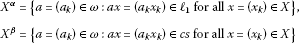and

where cs and bs are the sequence spaces of all convergent and bounded series, respectively .

We assume throughout that $p,q\ge 1$ with ${p}^{-1}+{q}^{-1}=1$ and denote the collection of all finite subsets of by .

## 3 The Fibonacci difference sequence spaces ${\ell }_{p}\left(\stackrel{ˆ}{F}\right)$ and ${\ell }_{\mathrm{\infty }}\left(\stackrel{ˆ}{F}\right)$

In this section, we define the Fibonacci band matrix $\stackrel{ˆ}{F}=\left({\stackrel{ˆ}{f}}_{nk}\right)$ and introduce the sequence spaces ${\ell }_{p}\left(\stackrel{ˆ}{F}\right)$ and ${\ell }_{\mathrm{\infty }}\left(\stackrel{ˆ}{F}\right)$, where $1\le p<\mathrm{\infty }$. Also, we present some inclusion theorems and construct the Schauder basis of the space ${\ell }_{p}\left(\stackrel{ˆ}{F}\right)$ for$1\le p<\mathrm{\infty }$.

Let ${f}_{n}$ be the n th Fibonacci number for every $n\in \mathbb{N}$. Then we define the infinite matrix $\stackrel{ˆ}{F}=\left({\stackrel{ˆ}{f}}_{nk}\right)$ by

Now, we introduce the Fibonacci difference sequence spaces ${\ell }_{p}\left(\stackrel{ˆ}{F}\right)$ and ${\ell }_{\mathrm{\infty }}\left(\stackrel{ˆ}{F}\right)$ as the set of all sequences such that their $\stackrel{ˆ}{F}$-transforms are in the space ${\ell }_{p}$ and ${\ell }_{\mathrm{\infty }}$, respectively, i.e.,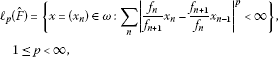and

${\ell }_{\mathrm{\infty }}\left(\stackrel{ˆ}{F}\right)=\left\{x=\left({x}_{n}\right)\in \omega :\underset{n\in \mathbb{N}}{sup}|\frac{{f}_{n}}{{f}_{n+1}}{x}_{n}-\frac{{f}_{n+1}}{{f}_{n}}{x}_{n-1}|<\mathrm{\infty }\right\}.$

With the notation of (1.2), the sequence spaces ${\ell }_{p}\left(\stackrel{ˆ}{F}\right)$ and ${\ell }_{\mathrm{\infty }}\left(\stackrel{ˆ}{F}\right)$ may be redefined by

${\ell }_{p}\left(\stackrel{ˆ}{F}\right)={\left({\ell }_{p}\right)}_{\stackrel{ˆ}{F}}\phantom{\rule{1em}{0ex}}\left(1\le p<\mathrm{\infty }\right)\phantom{\rule{1em}{0ex}}\text{and}\phantom{\rule{1em}{0ex}}{\ell }_{\mathrm{\infty }}\left(\stackrel{ˆ}{F}\right)={\left({\ell }_{\mathrm{\infty }}\right)}_{\stackrel{ˆ}{F}}.$
(3.1)

Define the sequence $y=\left({y}_{n}\right)$, which will be frequently used, by the $\stackrel{ˆ}{F}$-transform of a sequence $x=\left({x}_{n}\right)$, i.e.,

${y}_{n}={\stackrel{ˆ}{F}}_{n}\left(x\right)=\left\{\begin{array}{cc}\frac{{f}_{0}}{{f}_{1}}{x}_{0}={x}_{0}\hfill & \left(n=0\right),\hfill \\ \frac{{f}_{n}}{{f}_{n+1}}{x}_{n}-\frac{{f}_{n+1}}{{f}_{n}}{x}_{n-1}\hfill & \left(n\ge 1\right)\hfill \end{array}\phantom{\rule{1em}{0ex}}\left(n\in \mathbb{N}\right).$
(3.2)

Now, we may begin with the following theorem which is essential in the text.

Theorem 3.1 Let $1\le p\le \mathrm{\infty }$. Then ${\ell }_{p}\left(\stackrel{ˆ}{F}\right)$ is a BK-space with the norm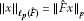, that is,

${\parallel x\parallel }_{{\ell }_{p}\left(\stackrel{ˆ}{F}\right)}={\left(\sum _{n}|{\stackrel{ˆ}{F}}_{n}\left(x\right){|}^{p}\right)}^{1/p}\phantom{\rule{1em}{0ex}}\left(1\le p<\mathrm{\infty }\right)$

and

${\parallel x\parallel }_{{\ell }_{\mathrm{\infty }}\left(\stackrel{ˆ}{F}\right)}=\underset{n\in \mathbb{N}}{sup}|{\stackrel{ˆ}{F}}_{n}\left(x\right)|.$

Proof Since (3.1) holds, ${\ell }_{p}$ and ${\ell }_{\mathrm{\infty }}$ are BK-spaces with respect to their natural norms and the matrix $\stackrel{ˆ}{F}$ is a triangle; Theorem 4.3.12 of Wilansky [, p.63] gives the fact that the spaces ${\ell }_{p}\left(\stackrel{ˆ}{F}\right)$ and ${\ell }_{\mathrm{\infty }}\left(\stackrel{ˆ}{F}\right)$ are BK-spaces with the given norms, where $1\le p<\mathrm{\infty }$. This completes the proof. □

Remark 3.2 One can easily check that the absolute property does not hold on the spaces ${\ell }_{p}\left(\stackrel{ˆ}{F}\right)$ and ${\ell }_{\mathrm{\infty }}\left(\stackrel{ˆ}{F}\right)$, that is, ${\parallel x\parallel }_{{\ell }_{p}\left(\stackrel{ˆ}{F}\right)}\ne {\parallel |x|\parallel }_{{\ell }_{p}\left(\stackrel{ˆ}{F}\right)}$ and ${\parallel x\parallel }_{{\ell }_{\mathrm{\infty }}\left(\stackrel{ˆ}{F}\right)}\ne {\parallel |x|\parallel }_{{\ell }_{\mathrm{\infty }}\left(\stackrel{ˆ}{F}\right)}$ for at least one sequence in the spaces ${\ell }_{p}\left(\stackrel{ˆ}{F}\right)$ and ${\ell }_{\mathrm{\infty }}\left(\stackrel{ˆ}{F}\right)$, and this shows that ${\ell }_{p}\left(\stackrel{ˆ}{F}\right)$ and ${\ell }_{\mathrm{\infty }}\left(\stackrel{ˆ}{F}\right)$ are the sequence spaces of non-absolute type, where $|x|=\left(|{x}_{k}|\right)$ and $1\le p<\mathrm{\infty }$.

Theorem 3.3 The Fibonacci difference sequence space ${\ell }_{p}\left(\stackrel{ˆ}{F}\right)$ of non-absolute type is linearly isomorphic to the space ${\ell }_{p}$, that is, ${\ell }_{p}\left(\stackrel{ˆ}{F}\right)\cong {\ell }_{p}$ for $1\le p\le \mathrm{\infty }$.

Proof To prove this, we should show the existence of a linear bijection between the spaces ${\ell }_{p}\left(\stackrel{ˆ}{F}\right)$ and ${\ell }_{p}$ for $1\le p\le \mathrm{\infty }$. Consider the transformation T defined, with the notation of (3.2), from ${\ell }_{p}\left(\stackrel{ˆ}{F}\right)$ to ${\ell }_{p}$ by $x\to y=Tx$. Then $Tx=y=\stackrel{ˆ}{F}x\in {\ell }_{p}$ for every $x\in {\ell }_{p}\left(\stackrel{ˆ}{F}\right)$. Also, the linearity of T is clear. Further, it is trivial that $x=0$ whenever $Tx=0$ and hence T is injective.

Furthermore, let $y=\left({y}_{k}\right)\in {\ell }_{p}$ for $1\le p\le \mathrm{\infty }$ and define the sequence $x=\left({x}_{k}\right)$ by

${x}_{k}=\sum _{j=0}^{k}\frac{{f}_{k+1}^{2}}{{f}_{j}{f}_{j+1}}{y}_{j}\phantom{\rule{1em}{0ex}}\left(k\in \mathbb{N}\right).$
(3.3)

Then, in the cases $1\le p<\mathrm{\infty }$ and $p=\mathrm{\infty }$, we get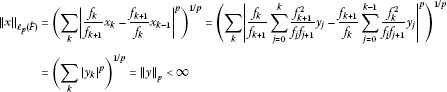and

${\parallel x\parallel }_{{\ell }_{\mathrm{\infty }}\left(\stackrel{ˆ}{F}\right)}=\underset{k\in \mathbb{N}}{sup}|{\stackrel{ˆ}{F}}_{k}\left(x\right)|={\parallel y\parallel }_{\mathrm{\infty }}<\mathrm{\infty },$

respectively. Thus, we have $x\in {\ell }_{p}\left(\stackrel{ˆ}{F}\right)$ ($1\le p\le \mathrm{\infty }$). Hence, T is surjective and norm preserving. Consequently, T is a linear bijection which shows that the spaces ${\ell }_{p}\left(\stackrel{ˆ}{F}\right)$ and ${\ell }_{p}$ are linearly isomorphic for $1\le p\le \mathrm{\infty }$. This concludes the proof. □

Now, we give some inclusion relations concerning the space ${\ell }_{p}\left(\stackrel{ˆ}{F}\right)$.

Theorem 3.4 The inclusion ${\ell }_{p}\subset {\ell }_{p}\left(\stackrel{ˆ}{F}\right)$ strictly holds for $1\le p\le \mathrm{\infty }$.

Proof To prove the validity of the inclusion ${\ell }_{p}\subset {\ell }_{p}\left(\stackrel{ˆ}{F}\right)$ for $1\le p\le \mathrm{\infty }$, it suffices to show the existence of a number $M>0$ such that ${\parallel x\parallel }_{{\ell }_{p}\left(\stackrel{ˆ}{F}\right)}\le M{\parallel x\parallel }_{p}$ for every $x\in {\ell }_{p}$.

Let $x\in {\ell }_{p}$ and $1. Since the inequalities $\frac{{f}_{k}}{{f}_{k+1}}\le 1$ and $\frac{{f}_{k+1}}{{f}_{k}}\le 2$ hold for every $k\in \mathbb{N}$, we obtain with the notation of (3.2),

$\sum _{k}|{\stackrel{ˆ}{F}}_{k}\left(x\right){|}^{p}\le \sum _{k}{2}^{p-1}\left(|{x}_{k}{|}^{p}+|2{x}_{k-1}{|}^{p}\right)\le {2}^{2p-1}\left(\sum _{k}|{x}_{k}{|}^{p}+\sum _{k}|{x}_{k-1}{|}^{p}\right)$

and

$\underset{k\in \mathbb{N}}{sup}|{\stackrel{ˆ}{F}}_{k}\left(x\right)|\le 3\underset{k\in \mathbb{N}}{sup}|{x}_{k}|,$

which together yield, as expected,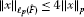(3.4)

for $1. Further, since the sequence $x=\left({x}_{k}\right)=\left({f}_{k+1}^{2}\right)=\left(1,{2}^{2},{3}^{2},{5}^{2},\dots \right)$ is in ${\ell }_{p}\left(\stackrel{ˆ}{F}\right)-{\ell }_{p}$, the inclusion ${\ell }_{p}\subset {\ell }_{p}\left(\stackrel{ˆ}{F}\right)$ is strict for $1. Similarly, one can easily prove that inequality (3.4) also holds in the case $p=1$, and so we omit the details. This completes the proof. □

Theorem 3.5 Neither of the spaces $b{v}_{p}$ and ${\ell }_{p}\left(\stackrel{ˆ}{F}\right)$ includes the other one, where $1\le p<\mathrm{\infty }$.

Proof Let $e=\left(1,1,1,\dots \right)$ and $x=\left({x}_{k}\right)=\left({f}_{k+1}^{2}\right)$. Then, since $\stackrel{ˆ}{F}x=\left(1,0,0,\dots \right)\in {\ell }_{p}$ and $\mathrm{\Delta }x=\left(1,{f}_{0}{f}_{3},{f}_{1}{f}_{4},\dots ,{f}_{k-1}{f}_{k+2},\dots \right)\notin {\ell }_{p}$, we conclude that x is in ${\ell }_{p}\left(\stackrel{ˆ}{F}\right)$ but not in $b{v}_{p}$. Now, consider the equation

$|\frac{{f}_{k}}{{f}_{k+1}}-\frac{{f}_{k+1}}{{f}_{k}}|=\frac{|{f}_{k}^{2}-{f}_{k+1}^{2}|}{{f}_{k}{f}_{k+1}}=\frac{|{\left(-1\right)}^{k}-{f}_{k}{f}_{k+1}|}{{f}_{k}{f}_{k+1}}\phantom{\rule{1em}{0ex}}\left(k\in \mathbb{N}\right).$

Then $|{\left(-1\right)}^{k}-{f}_{k}{f}_{k+1}|>{f}_{k}{f}_{k+1}$ whenever k is odd, which implies that the series ${\sum }_{k}|\frac{{f}_{k}}{{f}_{k+1}}-\frac{{f}_{k+1}}{{f}_{k}}{|}^{p}$ is not convergent, where $1\le p<\mathrm{\infty }$. Thus, $\stackrel{ˆ}{F}e=\left(\frac{{f}_{k}}{{f}_{k+1}}-\frac{{f}_{k+1}}{{f}_{k}}\right)$ is not in ${\ell }_{p}$ for $1\le p<\mathrm{\infty }$. Additionally, since $\mathrm{\Delta }e=\left(1,0,0,\dots \right)$, the sequence e is in ${\ell }_{p}$. Hence, the sequence spaces ${\ell }_{p}\left(\stackrel{ˆ}{F}\right)$ and $b{v}_{p}$ overlap but neither contains the other, as asserted. □

Theorem 3.6 If $1\le p, then ${\ell }_{p}\left(\stackrel{ˆ}{F}\right)\subset {\ell }_{s}\left(\stackrel{ˆ}{F}\right)$.

Proof Let $1\le p and $x\in {\ell }_{p}\left(\stackrel{ˆ}{F}\right)$. Then we obtain from Theorem 3.1 that $y\in {\ell }_{p}$, where y is the sequence given by (3.2). Thus, the well-known inclusion ${\ell }_{p}\subset {\ell }_{s}$ yields $y\in {\ell }_{s}$. This means that $x\in {\ell }_{s}\left(\stackrel{ˆ}{F}\right)$ and hence, the inclusion ${\ell }_{p}\left(\stackrel{ˆ}{F}\right)\subset {\ell }_{s}\left(\stackrel{ˆ}{F}\right)$ holds. This completes the proof. □

Now, we give a sequence of the points of the space ${\ell }_{p}\left(\stackrel{ˆ}{F}\right)$ which forms a basis for the space ${\ell }_{p}\left(\stackrel{ˆ}{F}\right)$ ($1\le p<\mathrm{\infty }$).

Theorem 3.7 Let $1\le p<\mathrm{\infty }$ and define the sequence ${c}^{\left(k\right)}\in {\ell }_{p}\left(\stackrel{ˆ}{F}\right)$ for every fixed $k\in \mathbb{N}$ by

${\left({c}^{\left(k\right)}\right)}_{n}=\left\{\begin{array}{cc}0\hfill & \left(n
(3.5)

Then the sequence ${\left({c}^{\left(k\right)}\right)}_{k=0}^{\mathrm{\infty }}$ is a basis for the space ${\ell }_{p}\left(\stackrel{ˆ}{F}\right)$, and every $x\in {\ell }_{p}\left(\stackrel{ˆ}{F}\right)$ has a unique representation of the form

$x=\sum _{k}{\stackrel{ˆ}{F}}_{k}\left(x\right){c}^{\left(k\right)}.$
(3.6)

Proof Let $1\le p<\mathrm{\infty }$. Then it is obvious by (3.5) that $\stackrel{ˆ}{F}\left({c}^{\left(k\right)}\right)={e}^{\left(k\right)}\in {\ell }_{p}$ ($k\in \mathbb{N}$) and hence ${c}^{\left(k\right)}\in {\ell }_{p}\left(\stackrel{ˆ}{F}\right)$ for all $k\in \mathbb{N}$.

Further, let $x\in {\ell }_{p}\left(\stackrel{ˆ}{F}\right)$ be given. For every non-negative integer m, we put

${x}^{\left(m\right)}=\sum _{k=0}^{m}{\stackrel{ˆ}{F}}_{k}\left(x\right){c}^{\left(k\right)}.$

Then we have that

$\stackrel{ˆ}{F}\left({x}^{\left(m\right)}\right)=\sum _{k=0}^{m}{\stackrel{ˆ}{F}}_{k}\left(x\right)\stackrel{ˆ}{F}\left({c}^{\left(k\right)}\right)=\sum _{k=0}^{m}{\stackrel{ˆ}{F}}_{k}\left(x\right){e}^{\left(k\right)}$

and hence

${\stackrel{ˆ}{F}}_{n}\left(x-{x}^{\left(m\right)}\right)=\left\{\begin{array}{cc}0\hfill & \left(0\le n\le m\right),\hfill \\ {\stackrel{ˆ}{F}}_{n}\left(x\right)\hfill & \left(n>m\right)\hfill \end{array}\phantom{\rule{1em}{0ex}}\left(n,m\in \mathbb{N}\right).$

Now, for any given $\epsilon >0$, there is a non-negative integer ${m}_{0}$ such that

$\sum _{n={m}_{0}+1}^{\mathrm{\infty }}|{\stackrel{ˆ}{F}}_{n}\left(x\right){|}^{p}\le {\left(\frac{\epsilon }{2}\right)}^{p}.$

Therefore, we have for every $m\ge {m}_{0}$ that

${\parallel x-{x}^{\left(m\right)}\parallel }_{{\ell }_{p}\left(\stackrel{ˆ}{F}\right)}={\left(\sum _{n=m+1}^{\mathrm{\infty }}|{\stackrel{ˆ}{F}}_{n}\left(x\right){|}^{p}\right)}^{1/p}\le {\left(\sum _{n={m}_{0}+1}^{\mathrm{\infty }}|{\stackrel{ˆ}{F}}_{n}\left(x\right){|}^{p}\right)}^{1/p}\le \frac{\epsilon }{2}<\epsilon ,$

which shows that ${lim}_{m\to \mathrm{\infty }}{\parallel x-{x}^{\left(m\right)}\parallel }_{{\ell }_{p}\left(\stackrel{ˆ}{F}\right)}=0$ and hence x is represented as in (3.6).

Finally, let us show the uniqueness of the representation (3.6) of $x\in {\ell }_{p}\left(\stackrel{ˆ}{F}\right)$. For this, suppose that $x={\sum }_{k}{\mu }_{k}\left(x\right){c}^{\left(k\right)}$. Since the linear transformation T defined from ${\ell }_{p}\left(\stackrel{ˆ}{F}\right)$ to ${\ell }_{p}$ in the proof of Theorem 3.3 is continuous, we have

${\stackrel{ˆ}{F}}_{n}\left(x\right)=\sum _{k}{\mu }_{k}\left(x\right){\stackrel{ˆ}{F}}_{n}\left({c}^{\left(k\right)}\right)=\sum _{k}{\mu }_{k}\left(x\right){\delta }_{nk}={\mu }_{n}\left(x\right)\phantom{\rule{1em}{0ex}}\left(n\in \mathbb{N}\right).$

Hence, the representation (3.6) of $x\in {\ell }_{p}\left(\stackrel{ˆ}{F}\right)$ is unique. This concludes the proof. □

## 4 The α-, β- and γ-duals of the space ${\ell }_{p}\left(\stackrel{ˆ}{F}\right)$

In this section, we determine the α-, β- and γ-duals of the sequence space ${\ell }_{p}\left(\stackrel{ˆ}{F}\right)$ of non-absolute type. Since the case $p=1$ can be proved by analogy, we omit the proof of that case and consider only the case $1 in the proof of Theorems 4.5 and 4.6, respectively.

The following known results  are fundamental for our investigation.

Lemma 4.1 $A=\left({a}_{nk}\right)\in \left({\ell }_{p},{\ell }_{1}\right)$ if and only if

$\underset{K\in \mathcal{F}}{sup}\sum _{k}|\sum _{n\in K}{a}_{nk}|<\mathrm{\infty },\phantom{\rule{1em}{0ex}}1

Lemma 4.2 $A=\left({a}_{nk}\right)\in \left({\ell }_{p},c\right)$ if and only if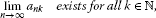(4.1)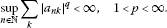(4.2)

Lemma 4.3 $A=\left({a}_{nk}\right)\in \left({\ell }_{\mathrm{\infty }},c\right)$ if and only if (4.1) holds and

$\underset{n\to \mathrm{\infty }}{lim}\sum _{k}|{a}_{nk}|=\sum _{k}|\underset{n\to \mathrm{\infty }}{lim}{a}_{nk}|.$
(4.3)

Lemma 4.4 $A=\left({a}_{nk}\right)\in \left({\ell }_{p},{\ell }_{\mathrm{\infty }}\right)$ if and only if (4.2) holds with $1.

Theorem 4.5 The α-dual of the space ${\ell }_{p}\left(\stackrel{ˆ}{F}\right)$ is the set

${\stackrel{ˆ}{d}}_{1}=\left\{a=\left({a}_{k}\right)\in \omega :\underset{K\in \mathcal{F}}{sup}\sum _{k}|\sum _{n\in K}\frac{{f}_{n+1}^{2}}{{f}_{k}{f}_{k+1}}{a}_{n}{|}^{q}<\mathrm{\infty }\right\},$

where $1.

Proof Let $1. For any fixed sequence $a=\left({a}_{n}\right)\in \omega$, we define the matrix $B=\left({b}_{nk}\right)$ by

${b}_{nk}=\left\{\begin{array}{cc}\frac{{f}_{n+1}^{2}}{{f}_{k}{f}_{k+1}}{a}_{n}\hfill & \left(0\le k\le n\right),\hfill \\ 0\hfill & \left(k>n\right)\hfill \end{array}$

for all $n,k\in \mathbb{N}$. Also, for every $x=\left({x}_{n}\right)\in \omega$, we put $y=\stackrel{ˆ}{F}x$. Then it follows by (3.2) that

${a}_{n}{x}_{n}=\sum _{k=0}^{n}\frac{{f}_{n+1}^{2}}{{f}_{k}{f}_{k+1}}{a}_{n}{y}_{k}={B}_{n}\left(y\right)\phantom{\rule{1em}{0ex}}\left(n\in \mathbb{N}\right).$
(4.4)

Thus, we observe by (4.4) that $ax=\left({a}_{n}{x}_{n}\right)\in {\ell }_{1}$ whenever $x\in {\ell }_{p}\left(\stackrel{ˆ}{F}\right)$ if and only if $By\in {\ell }_{1}$ whenever $y\in {\ell }_{p}$. Therefore, we derive by using Lemma 4.1 that

$\underset{K\in \mathcal{F}}{sup}\sum _{k}|\sum _{n\in K}\frac{{f}_{n+1}^{2}}{{f}_{k}{f}_{k+1}}{a}_{n}{|}^{q}<\mathrm{\infty },$

which implies that ${\left({\ell }_{p}\left(\stackrel{ˆ}{F}\right)\right)}^{\alpha }={\stackrel{ˆ}{d}}_{1}$. □

Theorem 4.6 Define the sets ${\stackrel{ˆ}{d}}_{2}$, ${\stackrel{ˆ}{d}}_{3}$ and ${\stackrel{ˆ}{d}}_{4}$ by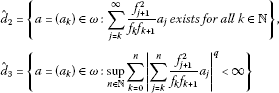and

${\stackrel{ˆ}{d}}_{4}=\left\{a=\left({a}_{k}\right)\in \omega :\underset{n\to \mathrm{\infty }}{lim}\sum _{k=0}^{n}|\sum _{j=k}^{n}\frac{{f}_{j+1}^{2}}{{f}_{k}{f}_{k+1}}{a}_{j}|=\sum _{k}|\sum _{j=k}^{\mathrm{\infty }}\frac{{f}_{j+1}^{2}}{{f}_{k}{f}_{k+1}}{a}_{j}|<\mathrm{\infty }\right\}.$

Then ${\left({\ell }_{p}\left(\stackrel{ˆ}{F}\right)\right)}^{\beta }={\stackrel{ˆ}{d}}_{2}\cap {\stackrel{ˆ}{d}}_{3}$ and ${\left({\ell }_{\mathrm{\infty }}\left(\stackrel{ˆ}{F}\right)\right)}^{\beta }={\stackrel{ˆ}{d}}_{2}\cap {\stackrel{ˆ}{d}}_{4}$, where $1.

Proof Let $a=\left({a}_{k}\right)\in \omega$ and consider the equality

$\sum _{k=0}^{n}{a}_{k}{x}_{k}=\sum _{k=0}^{n}{a}_{k}\left(\sum _{j=0}^{n}\frac{{f}_{k+1}^{2}}{{f}_{j}{f}_{j+1}}{y}_{j}\right)=\sum _{k=0}^{n}\left(\sum _{j=k}^{n}\frac{{f}_{j+1}^{2}}{{f}_{k}{f}_{k+1}}{a}_{j}\right){y}_{k}={D}_{n}\left(y\right),$
(4.5)

where $D=\left({d}_{nk}\right)$ is defined by

${d}_{nk}=\left\{\begin{array}{cc}\underset{j=k}{\sum ^{n}}\frac{{f}_{j+1}^{2}}{{f}_{k}{f}_{k+1}}{a}_{j}\hfill & \left(0\le k\le n\right),\hfill \\ 0\hfill & \left(k>n\right),\hfill \end{array}\phantom{\rule{1em}{0ex}}n,k\in \mathbb{N}.$

Then we deduce from Lemma 4.2 with (3.2) that $ax=\left({a}_{k}{x}_{k}\right)\in cs$ whenever $x=\left({x}_{k}\right)\in {\ell }_{p}\left(\stackrel{ˆ}{F}\right)$ if and only if $Dy\in c$ whenever $y=\left({y}_{k}\right)\in {\ell }_{p}$. Thus, $\left({a}_{k}\right)\in {\left({\ell }_{p}\left(\stackrel{ˆ}{F}\right)\right)}^{\beta }$ if and only if $\left({a}_{k}\right)\in {\stackrel{ˆ}{d}}_{2}$ and $\left({a}_{k}\right)\in {\stackrel{ˆ}{d}}_{3}$ by (4.1) and (4.2), respectively. Hence, ${\left({\ell }_{p}\left(\stackrel{ˆ}{F}\right)\right)}^{\beta }={\stackrel{ˆ}{d}}_{2}\cap {\stackrel{ˆ}{d}}_{3}$. It is clear that one can also prove the case $p=\mathrm{\infty }$ by the technique used in the proof of the case $1 with Lemma 4.3 instead of Lemma 4.2. So, we leave the detailed proof to the reader. □

Theorem 4.7 ${\left({\ell }_{p}\left(\stackrel{ˆ}{F}\right)\right)}^{\gamma }={\stackrel{ˆ}{d}}_{3}$, where $1.

Proof This result can be obtained from Lemma 4.4 by using (4.5). □

## 5 Some matrix transformations related to the sequence space ${\ell }_{p}\left(\stackrel{ˆ}{F}\right)$

In this section, we characterize the classes $\left({\ell }_{p}\left(\stackrel{ˆ}{F}\right),X\right)$, where $1\le p\le \mathrm{\infty }$ and X is any of the spaces ${\ell }_{\mathrm{\infty }}$, ${\ell }_{1}$, c and ${c}_{0}$.

For simplicity in notation, we write

${\stackrel{˜}{a}}_{nk}=\sum _{j=k}^{\mathrm{\infty }}\frac{{f}_{j+1}^{2}}{{f}_{k}{f}_{k+1}}{a}_{nj}$

for all $k,n\in \mathbb{N}$.

The following lemma is essential for our results.

Lemma 5.1 (see [, Theorem 4.1])

Let λ be an FK-space, U be a triangle, V be its inverse and μ be an arbitrary subset of ω. Then we have $A=\left({a}_{nk}\right)\in \left({\lambda }_{U},\mu \right)$ if and only if

${C}^{\left(n\right)}=\left({c}_{mk}^{\left(n\right)}\right)\in \left(\lambda ,c\right)\phantom{\rule{1em}{0ex}}\mathit{\text{for all}}\phantom{\rule{0.25em}{0ex}}n\in \mathbb{N}$

and

$C=\left({c}_{nk}\right)\in \left(\lambda ,\mu \right),$

where

${c}_{mk}^{\left(n\right)}=\left\{\begin{array}{cc}{\sum }_{j=k}^{m}{a}_{nj}{v}_{jk}\hfill & \left(0\le k\le m\right),\hfill \\ 0\hfill & \left(k>m\right)\hfill \end{array}$

and ${c}_{nk}={\sum }_{j=k}^{\mathrm{\infty }}{a}_{nj}{v}_{jk}$ for all $k,m,n\in \mathbb{N}$.

Now, we list the following conditions: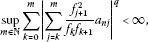(5.1)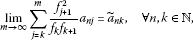(5.2)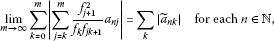(5.3)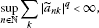(5.4)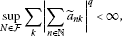(5.5)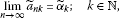(5.6)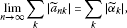(5.7)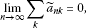(5.8)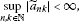(5.9)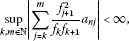(5.10)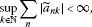(5.11)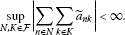(5.12)

Then, by combining Lemma 5.1 with the results in , we immediately derive the following results.

Theorem 5.2

1. (a)

$A=\left({a}_{nk}\right)\in \left({\ell }_{1}\left(\stackrel{ˆ}{F}\right),{\ell }_{\mathrm{\infty }}\right)$ if and only (5.2), (5.9) and (5.10) hold.

2. (b)

$A=\left({a}_{nk}\right)\in \left({\ell }_{1}\left(\stackrel{ˆ}{F}\right),c\right)$ if and only if (5.2), (5.6), (5.9) and (5.10) hold.

3. (c)

$A=\left({a}_{nk}\right)\in \left({\ell }_{1}\left(\stackrel{ˆ}{F}\right),{c}_{0}\right)$ if and only if (5.2), (5.6) with ${\stackrel{˜}{\alpha }}_{k}=0$, (5.9) and (5.10) hold.

4. (d)

$A=\left({a}_{nk}\right)\in \left({\ell }_{1}\left(\stackrel{ˆ}{F}\right),{\ell }_{1}\right)$ if and only (5.2), (5.10) and (5.11) hold.

Theorem 5.3 Let $1. Then we have

1. (a)

$A=\left({a}_{nk}\right)\in \left({\ell }_{p}\left(\stackrel{ˆ}{F}\right),{\ell }_{\mathrm{\infty }}\right)$ if and only if (5.1), (5.2) and (5.4) hold.

2. (b)

$A=\left({a}_{nk}\right)\in \left({\ell }_{p}\left(\stackrel{ˆ}{F}\right),c\right)$ if and only if (5.1), (5.2), (5.4) and (5.6) hold.

3. (c)

$A=\left({a}_{nk}\right)\in \left({\ell }_{p}\left(\stackrel{ˆ}{F}\right),{c}_{0}\right)$ if and only if (5.1), (5.2), (5.4) and (5.6) with ${\stackrel{˜}{\alpha }}_{k}=0$ hold.

4. (d)

$A=\left({a}_{nk}\right)\in \left({\ell }_{p}\left(\stackrel{ˆ}{F}\right),{\ell }_{1}\right)$ if and only if (5.1), (5.2) and (5.5) hold.

Theorem 5.4

1. (a)

$A=\left({a}_{nk}\right)\in \left({\ell }_{\mathrm{\infty }}\left(\stackrel{ˆ}{F}\right),{\ell }_{\mathrm{\infty }}\right)$ if and only (5.2), (5.3) and (5.4) with $q=1$ hold.

2. (b)

$A=\left({a}_{nk}\right)\in \left({\ell }_{\mathrm{\infty }}\left(\stackrel{ˆ}{F}\right),c\right)$ if and only (5.2), (5.3), (5.6) and (5.7) hold.

3. (c)

$A=\left({a}_{nk}\right)\in \left({\ell }_{\mathrm{\infty }}\left(\stackrel{ˆ}{F}\right),{c}_{0}\right)$ if and only (5.2), (5.3) and (5.8) hold.

4. (d)

$A=\left({a}_{nk}\right)\in \left({\ell }_{\mathrm{\infty }}\left(\stackrel{ˆ}{F}\right),{\ell }_{1}\right)$ if and only (5.2), (5.3) and (5.12) hold.

## 6 Some geometric properties of the space ${\ell }_{p}\left(\stackrel{ˆ}{F}\right)$ ($1)

In this section, we study some geometric properties of the space ${\ell }_{p}\left(\stackrel{ˆ}{F}\right)$ for $1.

For these properties, we refer to [3, 3947].

A Banach space X is said to have the Banach-Saks property if every bounded sequence $\left({x}_{n}\right)$ in X admits a subsequence $\left({z}_{n}\right)$ such that the sequence $\left\{{t}_{k}\left(z\right)\right\}$ is convergent in the norm in X , where

${t}_{k}\left(z\right)=\frac{1}{k+1}\left({z}_{0}+{z}_{1}+\cdots +{z}_{k}\right)\phantom{\rule{1em}{0ex}}\left(k\in \mathbb{N}\right).$
(6.1)

A Banach space X is said to have the weak Banach-Saks property whenever, given any weakly null sequence $\left({x}_{n}\right)\subset X$, there exists a subsequence $\left({z}_{n}\right)$ of $\left({x}_{n}\right)$ such that the sequence $\left\{{t}_{k}\left(z\right)\right\}$ is strongly convergent to zero.

In , García-Falset introduces the following coefficient:

$R\left(X\right)=sup\left\{\underset{n\to \mathrm{\infty }}{liminf}\parallel {x}_{n}-x\parallel :\left({x}_{n}\right)\subset B\left(X\right),{x}_{n}\stackrel{w}{\to }0,x\in B\left(X\right)\right\},$
(6.2)

where $B\left(X\right)$ denotes the unit ball of X.

Remark 6.1 A Banach space X with $R\left(X\right)<2$ has the weak fixed point property .

Let $1. A Banach space is said to have the Banach-Saks type p or the property ${\left(BS\right)}_{p}$ if every weakly null sequence $\left({x}_{k}\right)$ has a subsequence $\left({x}_{{k}_{l}}\right)$ such that for some $C>0$,

$\parallel \sum _{l=0}^{n}{x}_{{k}_{l}}\parallel
(6.3)

for all $n\in \mathbb{N}$ ( see ).

Now, we may give the following results related to some geometric properties, mentioned above, of the space ${\ell }_{p}\left(\stackrel{ˆ}{F}\right)$, where $1.

Theorem 6.2 Let $1. Then the space ${\ell }_{p}\left(\stackrel{ˆ}{F}\right)$ has the Banach-Saks type p.

Proof Let $\left({\epsilon }_{n}\right)$ be a sequence of positive numbers for which $\sum {\epsilon }_{n}\le 1/2$, and also let $\left({x}_{n}\right)$ be a weakly null sequence in $B\left({\ell }_{p}\left(\stackrel{ˆ}{F}\right)\right)$. Set ${z}_{0}={x}_{0}=0$ and ${z}_{1}={x}_{{n}_{1}}={x}_{1}$. Then there exists ${m}_{1}\in \mathbb{N}$ such that

${\parallel \sum _{i={m}_{1}+1}^{\mathrm{\infty }}{z}_{1}\left(i\right){e}^{\left(i\right)}\parallel }_{{\ell }_{p}\left(\stackrel{ˆ}{F}\right)}<{\epsilon }_{1}.$
(6.4)

Since $\left({x}_{n}\right)$ being a weakly null sequence implies ${x}_{n}\to 0$ coordinatewise, there is an ${n}_{2}\in \mathbb{N}$ such that

${\parallel \sum _{i=0}^{{m}_{1}}{x}_{n}\left(i\right){e}^{\left(i\right)}\parallel }_{{\ell }_{p}\left(\stackrel{ˆ}{F}\right)}<{\epsilon }_{1},$

when $n\ge {n}_{2}$. Set ${z}_{2}={x}_{{n}_{2}}$. Then there exists an ${m}_{2}>{m}_{1}$ such that

${\parallel \sum _{i={m}_{2}+1}^{\mathrm{\infty }}{z}_{2}\left(i\right){e}^{\left(i\right)}\parallel }_{{\ell }_{p}\left(\stackrel{ˆ}{F}\right)}<{\epsilon }_{2}.$

Again using the fact that ${x}_{n}\to 0$ coordinatewise, there exists an ${n}_{3}\ge {n}_{2}$ such that

${\parallel \sum _{i=0}^{{m}_{2}}{x}_{n}\left(i\right){e}^{\left(i\right)}\parallel }_{{\ell }_{p}\left(\stackrel{ˆ}{F}\right)}<{\epsilon }_{2},$

when $n\ge {n}_{3}$.

If we continue this process, we can find two increasing subsequences $\left({m}_{i}\right)$ and $\left({n}_{i}\right)$ such that

${\parallel \sum _{i=0}^{{m}_{j}}{x}_{n}\left(i\right){e}^{\left(i\right)}\parallel }_{{\ell }_{p}\left(\stackrel{ˆ}{F}\right)}<{\epsilon }_{j}$

for each $n\ge {n}_{j+1}$ and

${\parallel \sum _{i={m}_{j}+1}^{\mathrm{\infty }}{z}_{j}\left(i\right){e}^{\left(i\right)}\parallel }_{{\ell }_{p}\left(\stackrel{ˆ}{F}\right)}<{\epsilon }_{j},$

where ${b}_{j}={x}_{{n}_{j}}$. Hence,

$\begin{array}{rcl}{\parallel \sum _{j=0}^{n}{z}_{j}\parallel }_{{\ell }_{p}\left(\stackrel{ˆ}{F}\right)}& =& {\parallel \sum _{j=0}^{n}\left(\sum _{i=0}^{{m}_{j-1}}{z}_{j}\left(i\right){e}^{\left(i\right)}+\sum _{i={m}_{j-1}+1}^{{m}_{j}}{z}_{j}\left(i\right){e}^{\left(i\right)}+\sum _{i={m}_{j}+1}^{\mathrm{\infty }}{z}_{j}\left(i\right){e}^{\left(i\right)}\right)\parallel }_{{\ell }_{p}\left(\stackrel{ˆ}{F}\right)}\\ \le & {\parallel \sum _{j=0}^{n}\left(\sum _{i={m}_{j-1}+1}^{{m}_{j}}{z}_{j}\left(i\right){e}^{\left(i\right)}\right)\parallel }_{{\ell }_{p}\left(\stackrel{ˆ}{F}\right)}+2\sum _{j=0}^{n}{\epsilon }_{j}.\end{array}$

On the other hand, it can be seen that ${\parallel x\parallel }_{{\ell }_{p}\left(\stackrel{ˆ}{F}\right)}<1$. Therefore, we have that

$\begin{array}{rcl}{\parallel \sum _{j=0}^{n}\left(\sum _{i={m}_{j-1}+1}^{{m}_{j}}{z}_{j}\left(i\right){e}^{\left(i\right)}\right)\parallel }_{{\ell }_{p}\left(\stackrel{ˆ}{F}\right)}^{p}& =& \sum _{j=0}^{n}\sum _{i={m}_{j-1}+1}^{{m}_{j}}|\frac{{f}_{i}}{{f}_{i+1}}{z}_{j}\left(i\right)-\frac{{f}_{i+1}}{{f}_{i}}{z}_{j}\left(i-1\right){|}^{p}\\ \le & \sum _{j=0}^{n}\sum _{i=0}^{\mathrm{\infty }}|\frac{{f}_{i}}{{f}_{i+1}}{z}_{j}\left(i\right)-\frac{{f}_{i+1}}{{f}_{i}}{z}_{j}\left(i-1\right){|}^{p}\\ \le & \left(n+1\right).\end{array}$

Hence, we obtain

$\parallel \sum _{j=0}^{n}\left(\sum _{i={m}_{j-1}+1}^{{m}_{j}}{z}_{j}\left(i\right){e}^{\left(i\right)}\right)\parallel \le {\left(n+1\right)}^{1/p}.$

By using the fact that $1\le {\left(n+1\right)}^{1/p}$ for all $n\in \mathbb{N}$ and $1, we have

${\parallel \sum _{j=0}^{n}{z}_{j}\parallel }_{{\ell }_{p}\left(\stackrel{ˆ}{F}\right)}\le {\left(n+1\right)}^{1/p}+1\le 2{\left(n+1\right)}^{1/p}.$

Hence, ${\ell }_{p}\left(\stackrel{ˆ}{F}\right)$ has the Banach-Saks type p. This concludes the proof. □

Remark 6.3 Note that $R\left({\ell }_{p}\left(\stackrel{ˆ}{F}\right)\right)=R\left({\ell }_{p}\right)={2}^{1/p}$ since ${\ell }_{p}\left(\stackrel{ˆ}{F}\right)$ is linearly isomorphic to ${\ell }_{p}$.

Hence, by Remarks 6.1 and 6.3, we have the following theorem.

Theorem 6.4 The space ${\ell }_{p}\left(\stackrel{ˆ}{F}\right)$ has the weak fixed point property, where $1.

## References

1. Aydın C, Başar F:Some new sequence spaces which include the spaces ${\ell }_{p}$ and ${\ell }_{\mathrm{\infty }}$. Demonstr. Math. 2005, 38(3):641–656.

2. Mursaleen M, Başar F, Altay B:On the Euler sequence spaces which include the spaces ${\ell }_{p}$ and ${\ell }_{\mathrm{\infty }}$ II. Nonlinear Anal. TMA 2006, 65(3):707–717. 10.1016/j.na.2005.09.038

3. Savaş E, Karakaya V, Şimşek N:Some $\ell \left(p\right)$-type new sequence spaces and their geometric properties. Abstr. Appl. Anal. 2009., 2009: Article ID 696971

4. Başar F, Altay B: On the space of sequences of p -bounded variation and related matrix mappings. Ukr. Math. J. 2003, 55: 136–147. 10.1023/A:1025080820961

5. Altay B, Başar F:Generalization of the sequence space $\ell \left(p\right)$ derived by weighted mean. J. Math. Anal. Appl. 2007, 330: 174–185. 10.1016/j.jmaa.2006.07.050

6. Mursaleen M, Noman AK:On some new sequence spaces of non-absolute type related to the spaces ${\ell }_{p}$ and ${\ell }_{\mathrm{\infty }}$ I. Filomat 2011, 25(2):33–51. 10.2298/FIL1102033M

7. Aydın C, Başar F:Some generalizations of the sequence spaces ${a}_{r}^{p}$. Iran. J. Sci. Technol., Trans. A, Sci. 2006, 30(A2):175–190.

8. Savaş E: Matrix transformations of some generalized sequence spaces. J. Orissa Math. Soc. 1985, 4(1):37–51.

9. Savaş E: Matrix transformations and absolute almost convergence. Bull. Inst. Math. Acad. Sin. 1987, 15(3):345–355.

10. Savaş E: Matrix transformations between some new sequence spaces. Tamkang J. Math. 1988, 19(4):75–80.

11. Savaş E: Matrix transformations and almost convergence. Math. Stud. 1991, 59(1–4):170–176.

12. Savaş E, Mursaleen M: Matrix transformations in some sequence spaces. Istanb. Üniv. Fen Fak. Mat. Derg. 1993, 52: 1–5.

13. Kızmaz H: On certain sequence spaces. Can. Math. Bull. 1981, 24(2):169–176. 10.4153/CMB-1981-027-5

14. Altay B, Başar F:The matrix domain and the fine spectrum of the difference operator Δ on the sequence space ${\ell }_{p}$, ($0). Commun. Math. Anal. 2007, 2(2):1–11.

15. Çolak R, Et M, Malkowsky E: Some Topics of Sequence Spaces. Fırat Univ. Press, Elazığ; 2004:1–63. ISBN:975–394–0386–6

16. Ahmad ZU, Mursaleen M: Köthe-Toeplitz duals of some new sequence spaces and their matrix maps. Publ. Inst. Math. (Belgr.) 1987, 42: 57–61.

17. Malkowsky E: Absolute and ordinary Köthe-Toeplitz duals of some sets of sequences and matrix transformations. Publ. Inst. Math. (Belgr.) 1989, 46(60):97–103.

18. Simons S:The sequence spaces $\ell \left({p}_{v}\right)$ and $m\left({p}_{v}\right)$. Proc. Lond. Math. Soc. 1965, 3(15):422–436.

19. Maddox IJ: Continuous and Köthe-Toeplitz duals of certain sequence spaces. Proc. Camb. Philos. Soc. 1965, 65: 431–435.

20. Altay B, Başar F, Mursaleen M: Some generalizations of the space $b{v}_{p}$ of p -bounded variation sequences. Nonlinear Anal. TMA 2008, 68: 273–287. 10.1016/j.na.2006.10.047

21. Kirişçi M, Başar F: Some new sequence spaces derived by the domain of generalized difference matrix. Comput. Math. Appl. 2010, 60: 1299–1309. 10.1016/j.camwa.2010.06.010

22. Sönmez A: Some new sequence spaces derived by the domain of the triple band matrix. Comput. Math. Appl. 2011, 62(2):641–650. 10.1016/j.camwa.2011.05.045

23. Choudhary B, Mishra SK: A note on Köthe-Toeplitz duals of certain sequence spaces and their matrix transformations. Int. J. Math. Math. Sci. 1995, 18(4):681–688. 10.1155/S0161171295000871

24. Sarıgöl MA: On difference sequence spaces. J. Karadeniz Tech. Univ., Fac. Arts Sci., Ser. Math.-Phys. 1987, 10: 63–71.

25. Et M: On some difference sequence spaces. Turk. J. Math. 1993, 17: 18–24.

26. Mursaleen M: Generalized spaces of difference sequences. J. Math. Anal. Appl. 1996, 203(3):738–745. 10.1006/jmaa.1996.0409

27. Mishra SK: Matrix maps involving certain sequence spaces. Indian J. Pure Appl. Math. 1993, 24(2):125–132.

28. Gaur AK, Mursaleen M: Difference sequence spaces. Int. J. Math. Math. Sci. 1998, 21(4):701–706. 10.1155/S0161171298000970

29. Malkowsky E, Mursaleen M:Some matrix transformations between the difference sequence spaces $\mathrm{\Delta }c\mathrm{0}\left(p\right)$, $\mathrm{\Delta }c\left(p\right)$ and $\mathrm{\Delta }{\ell }_{\mathrm{\infty }}\left(p\right)$. Filomat 2001, 15: 353–363.

30. Mursaleen M, Gaur AK, Saifi AH: Some new sequence spaces and their duals and matrix transformations. Bull. Calcutta Math. Soc. 1996, 88(3):207–212.

31. Sönmez A: Almost convergence and triple band matrix. Math. Comput. Model. 2012. doi:10.1016/j.mcm.2011.11.079

32. Başar F, Kirişçi M: Almost convergence and generalized difference matrix. Comput. Math. Appl. 2011, 61(3):602–611. 10.1016/j.camwa.2010.12.006

33. Et M, Çolak R: On some generalized difference sequence spaces. Soochow J. Math. 1995, 21: 377–386.

34. Et M, Esi A: On Köthe-Toeplitz duals of generalized difference sequence spaces. Bull. Malays. Math. Soc. 2000, 23: 25–32.

35. Koshy T: Fibonacci and Lucas Numbers with Applications. Wiley, New York; 2001.

36. Başar F: Summability Theory and Its Applications. Bentham Science Publishers, İstanbul; 2012. (e-books, Monographs, to appear)

37. Wilansky A North-Holland Mathematics Studies 85. In Summability Through Functional Analysis. Elsevier, Amsterdam; 1984.

38. Stieglitz M, Tietz H: Matrix transformationen von folgenräumen eine ergebnisübersicht. Math. Z. 1977, 154: 1–16. 10.1007/BF01215107

39. Demiriz S, Çakan C: Some topological and geometrical properties of a new difference sequence space. Abstr. Appl. Anal. 2011., 2011: Article ID 213878

40. Mursaleen M, Başar F, Altay B:On the Euler sequence spaces which include the spaces ${\ell }_{p}$ and ${\ell }_{\mathrm{\infty }}$ II. Nonlinear Anal. TMA 2006, 65(3):707–717. 10.1016/j.na.2005.09.038

41. Mursaleen M, Çolak R, Et M: Some geometric inequalities in a new Banach sequence space. J. Inequal. Appl. 2007., 2007: Article ID 86757

42. Diestel J Graduate Texts in Mathematics 92. In Sequence and Series in Banach Spaces. Springer, New York; 1984.

43. García-Falset J: Stability and fixed points for nonexpansive mappings. Houst. J. Math. 1994, 20(3):495–506.

44. García-Falset J: The fixed point property in Banach spaces with the NUS-property. J. Math. Anal. Appl. 1997, 215(2):532–542. 10.1006/jmaa.1997.5657

45. Knaust H: Orlicz sequence spaces of Banach-Saks type. Arch. Math. 1992, 59(6):562–565. 10.1007/BF01194848

46. Kananthai A, Mursaleen M, Sanhan W, Suantai S: On property (H) and rotundity of difference sequence spaces. J. Nonlinear Convex Anal. 2002, 3(3):401–409.

47. Mursaleen M:On some geometric properties of a sequence space related to ${\ell }^{p}$. Bull. Aust. Math. Soc. 2003, 67: 343–347. 10.1017/S0004972700033803

## Acknowledgements

The authors wish to thank the referee for his/her valuable suggestions, which improved the paper considerably.

## Author information

Authors

### Corresponding author

Correspondence to Emrah Evren Kara.

### Competing interests

The author declares that they have no competing interests.

## Rights and permissions

Reprints and Permissions

Kara, E.E. Some topological and geometrical properties of new Banach sequence spaces. J Inequal Appl 2013, 38 (2013). https://doi.org/10.1186/1029-242X-2013-38

• Accepted:

• Published:

• DOI: https://doi.org/10.1186/1029-242X-2013-38

### Keywords

• sequence spaces
• Fibonacci numbers
• difference matrix
• α-, β-, γ-duals
• matrix transformations
• fixed point property
• Banach-Saks type p